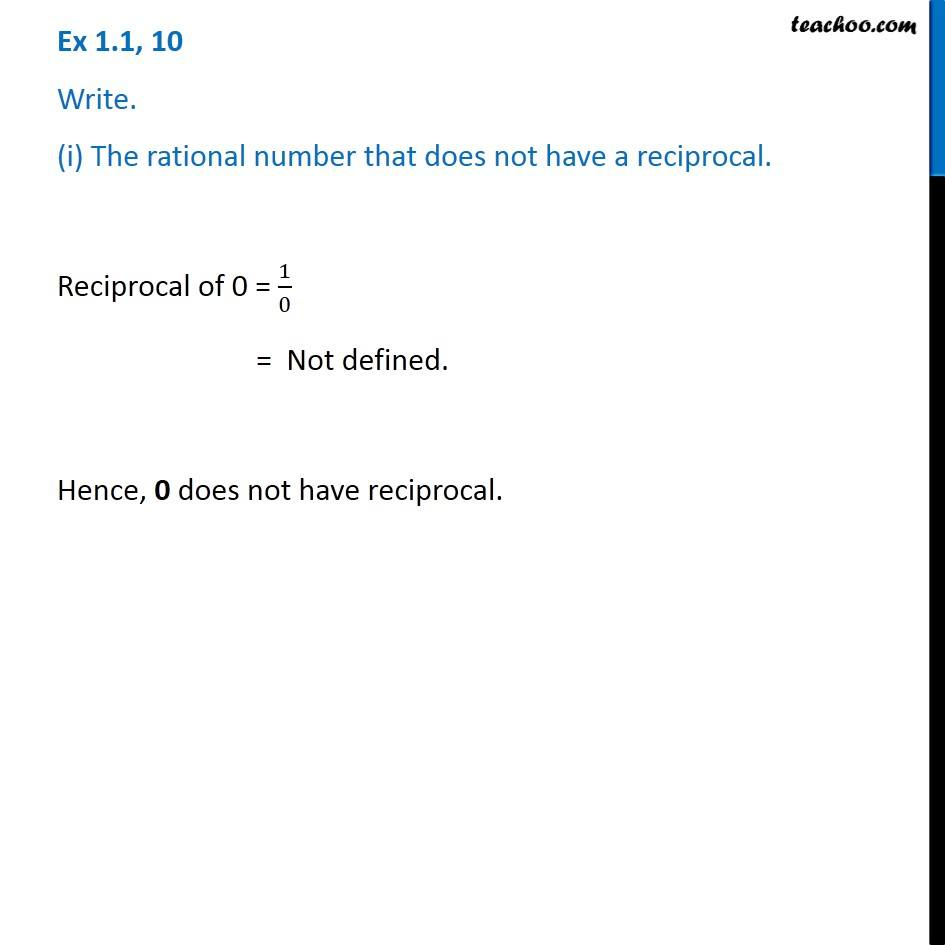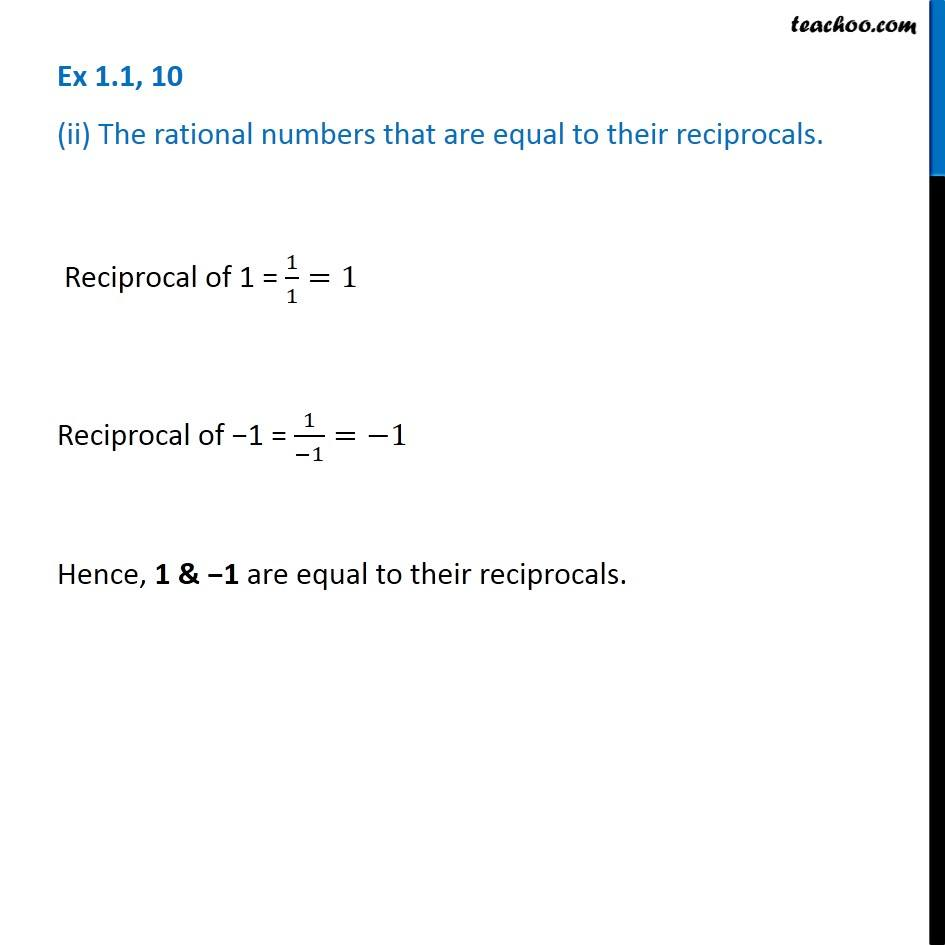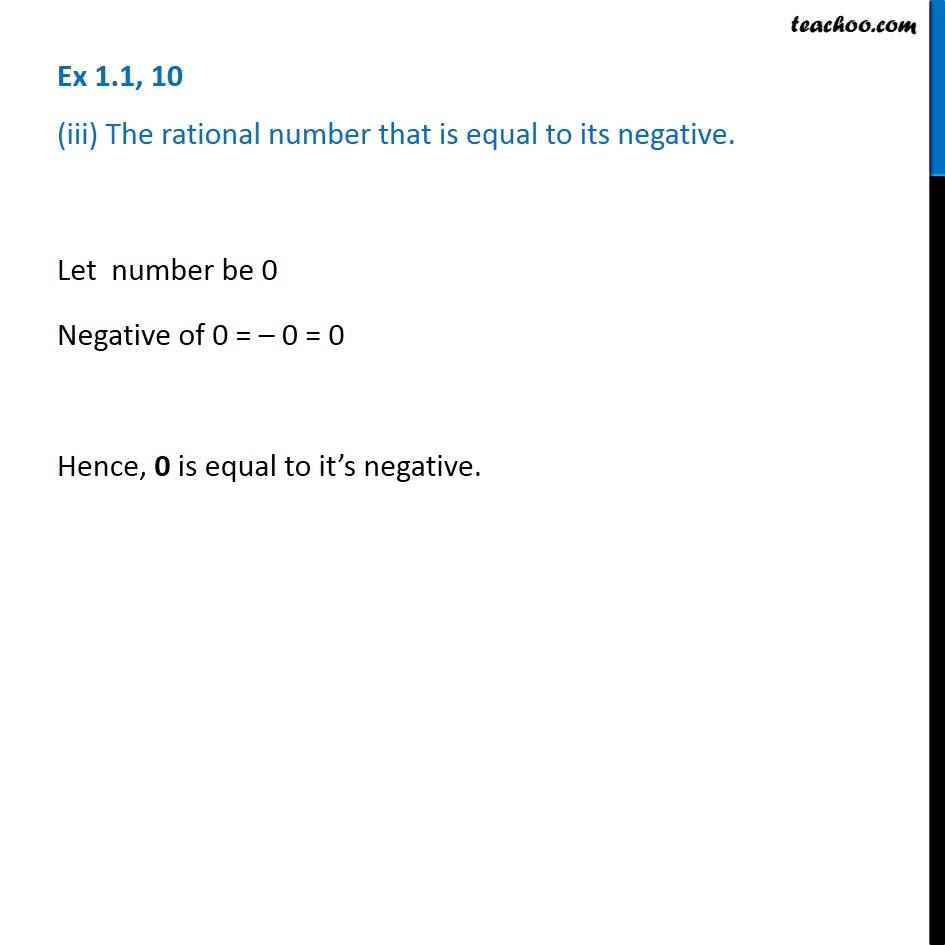Subscribe to our Youtube Channel - https://you.tube/teachoo

1. Chapter 1 Class 8 Rational Numbers
2. Serial order wise
3. Ex 1.1

Transcript

Ex 1.1, 10 Write. (i) The rational number that does not have a reciprocal. Reciprocal of 0 = 1/0 = Not defined. Hence, 0 does not have reciprocal. Ex 1.1, 10 (ii) The rational numbers that are equal to their reciprocals. Reciprocal of 1 = 1/1=1 Reciprocal of 1 = 1/( 1)= 1 Hence, 1 & 1 are equal to their reciprocals. Ex 1.1, 10 (iii) The rational number that is equal to its negative. Let number be 0 Negative of 0 = 0 = 0 Hence, 0 is equal to it s negative.

Ex 1.1